﻿ Crystal parameters description,Crystal Project Name

## 深圳市东泰河科技有限公司

Shenzhen TH Technology Co. Ltd.手机版全球咨询热线： 0755-27872782

# Crystal parameters description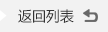来源：泰河 查看手机网址

About Crystal parameters description,Crystal Project Name

AT Cut Crystals

For precise frequency control in radio and line communication systems, quartz crystal resonators have proved indispensable. The material properties of crystalline quartz are such that quartz resonators display stableness and Q factors that cannot be matched by other types of resonator over the frequency range from 1 MHz to 200 MHz.

Equivalent Circuit

Fig-1 shows the conventionally accepted equivalent circuit of a crystal resonator at a frequency near its main mode of vibration. The inductance LI reiperesents the vibrating mass, the series capacitance CL the compliance of the quartz element and the resistance Rl the internal frication of the element, mechanical losses in the mounting system and acoustical losses to the surrounding environment.

The shunt capacitance Co is made up of the static capacitance between the electrodes, togettier with stray capacitances of the mounting system.

There are two zero-phase frequencies associated with this simple circuit, one is at series resonance fs, another at antiresonance fa. When used in an oscillator, crystal units will operate at any frequency within the broken lines of Fig-2 as determined by the phase of the maintaining circuit.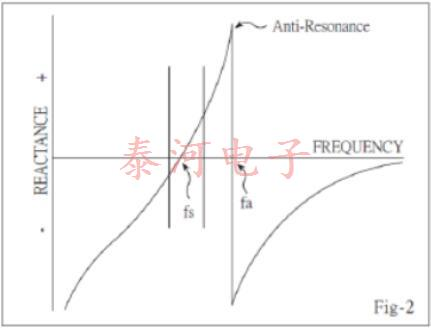By changing of this reactive condition, the crystal frequency may be trimmed in a limited extent. The degree to which this frequency may be varied (frequency pulling) is inversely proportional to the capacitance ratio r(C /Ci).

Many practical oscillator circuits make use of a load capacitor CL in series or parallel with the crystal, either in order to provide a means for final frequency adjustment, or perhaps for modulation or temperature compensation purposes. For the crystal load capacitance. We looking into the circuit through the two crystal terminals, the load capacitance need to specified when the crystal is paralleled mode, crystal load capacitance is calculated as below:

Frequency Pulling

In many applications a variable capacitor (trimmer) is used as the load reactive element to adjust the frequency. The fractional frequency range available between specified values of this load reactive element is called the pulling range (PR.) and it can be calculated by using the following formula:Sensitivity

A useful parameter to the design engineer is the pulling sensitivity (S) at a specified value of load capacitance. It is defined as the incremental fractional frequency change for an incremental change in load capacitance. It is normally expressed in ppm/pF (10-6/pF) and can be calculated from the formula: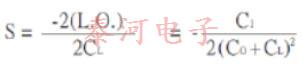It is very important to define the mean load capacitance to enable the actual crystal frequency be set within the tolerances of the specified nominal frequency. It is also important to use, wherever possible, standard values of load capacitance; for example:20pF, 30pF.

Fig-3 shows the relationship between LO.; P.R. and S.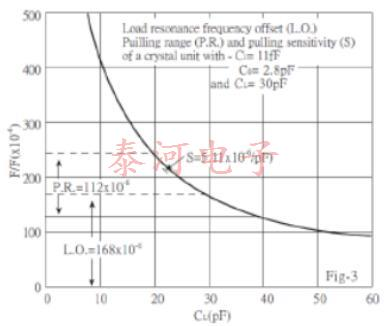Frequency Pulling Calculation

An approximation to the pulling for any crystal can be calculated from this simple formula: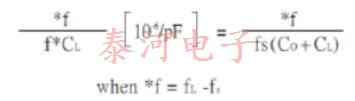Resistance

The equivalent circuit of the crystal has one other important parameter: This is Ri, the motional resistance. This parameter controls the Q of the crystal unit and will define the level of oscillation in any maintaining circuit. The load resonance for a given crystal unit depends upon the load capacitance with which that unit is intended to operate. The frequency of oscillation is the same in either series or parallel connection of the load capacitance.

If the external capacitance is designated the load resonance resistance may be calculated as follows: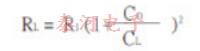The equivalent shunt or parallel resistance at load resonance frequency is approximately: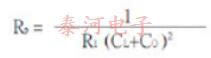It should be remembered that Ri does not change thus the effective parameters of any user network can be readily calculated.

Frequency Temperature Characteristics

The AT-cut crystal has a frequency temperature characteristic which may be described by a cubic function of temperature. This characteristic can be precisely controlled by small variations in the exact angle at which the crystal blank is cut from the original quartz bar. Fig,4 illustrates some typical cases. This cubic behaviour is in contrast to most other crystal cuts, which have parabolic temperature characteristics.

As a consequence, the AT-cut is generally the best choice when specifying a unit to operate over a wide temperature range, and is available in a range of frequencies from 1 to 200 MHz.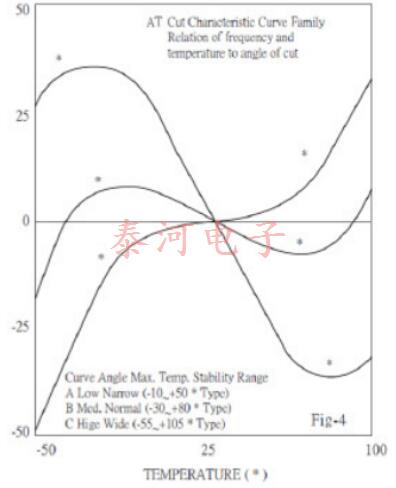#### “推荐阅读”

【本文标签】：Crystal parameters description Crystal Project Name
【责任编辑】：泰河版权所有：http://www.smdcrystal.com转载请注明出处